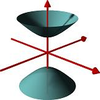#### You may also like### A Close Match

Can you massage the parameters of these curves to make them match as closely as possible?### Prime Counter

A short challenge concerning prime numbers.### The Right Volume

Can you rotate a curve to make a volume of 1?

# Seriesly

##### Age 16 to 18 ShortChallenge Level
This task previously appeared on the main NRICH site, with solutions as follows:

Thank you for these solutions to Shahnawaz Abdullah; Daniel, Liceo Scientifico Copernico, Torino, Italy; Anderthan, Saratoga High School; Andrei, School 205, Bucharest, Romania; David, Queen Mary's Grammar School, Walsall; Paddy, Peter, Greshams School, Holt, Norfolk; Ngoc Tran, Nguyen Truong To High School (Vietnam); Chris, St. Bees School; Dorothy, Madras College; A Ji and Hyeyoun, St. Paul's Girls' School; and Yatir from Israel.

To prove that $k \times k! = (k+1)! - k!$. If we take $k!$ out as a factor from the right hand side of the equation, we are left with $k! \times ((k+1)-1)$ which simplifies to $k \times k!$, as required.

Now we sum the series $1 \times 1!+.....n \times n!$ As we have proved, $n \times n!$ is equal to $(n+1)! - n!$ and therefore $(n-1) \times (n-1)!$ is equal to $(n-1+1)! - (n-1)!$ which simplifies to $n! - (n-1)!$.

If we add the two results, we find that $n!$ cancels. If we sum the series from 1 to $n$, we find that all of the terms cancel except for $(n+1)!$ and $-(1!)$. Thus the sum of all numbers of the form $r \times r!$ from $1$ to $n$ is equal to $(n+1)! - 1$.